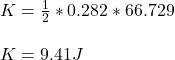Question

A hollow spherical shell has mass 8.35 kg and radius 0.225 m. It is initially at rest and then rotates about a stationary axis that lies along a diameter with a constant acceleration of 0.885 rad/s^2.

Required:
What is the kinetic energy of the shell after it has turned through 6.00 rev?

1.K = 9.41 J

Explanation:

The kinetic energy of a spherical shell is given as: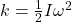where I = moment of inertia and

ω = angular velocity

Let us find I:

For a hollow sphere: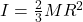where M = mass = 8.35 kg

R = radius = 0.225 m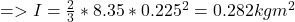Let us find ω:

Since angular acceleration is constant: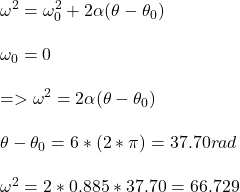Therefore, its kinetic energy is: